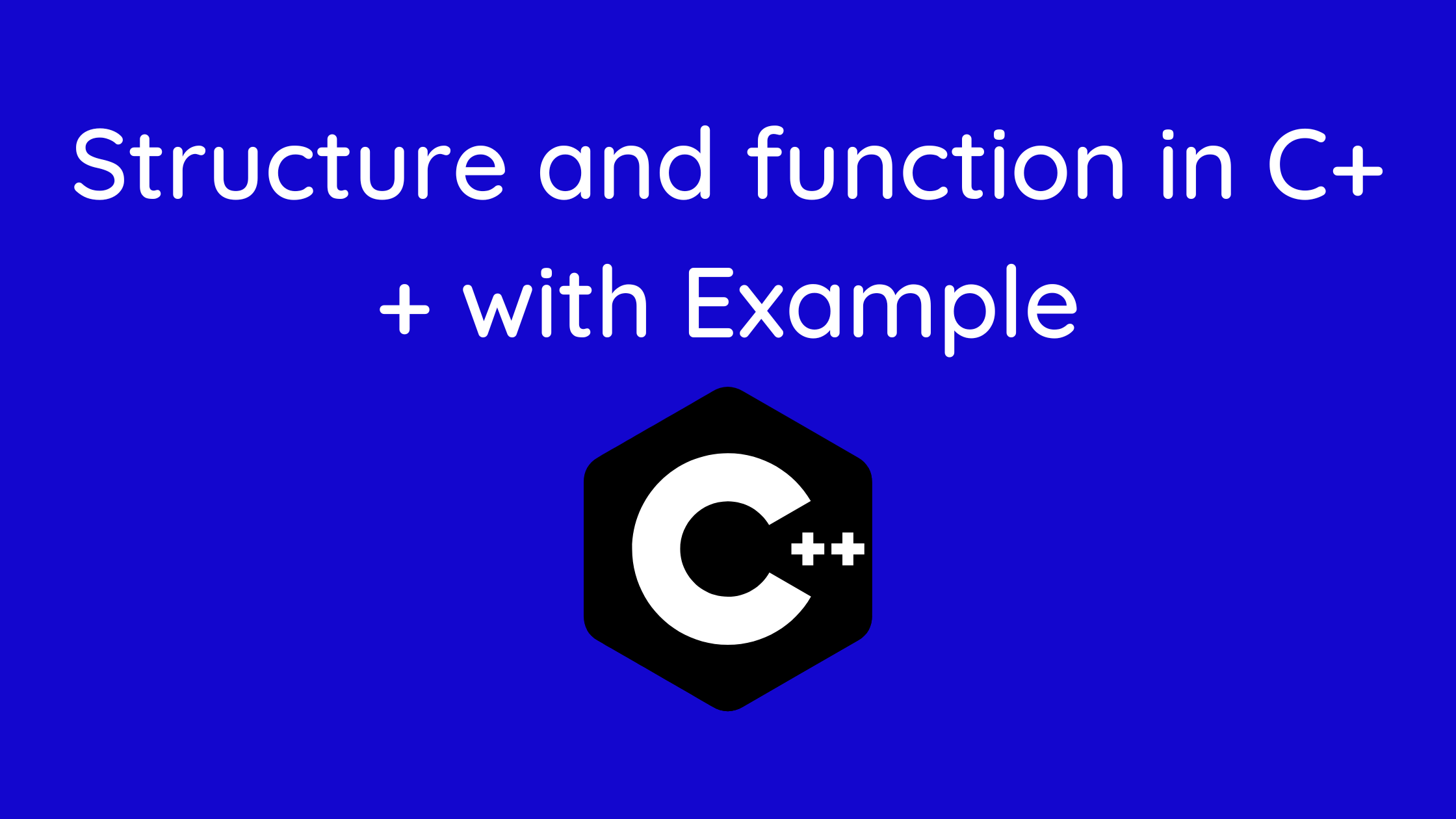## Structure and Function In C++

In this tutorial of structure and function, You’ll discover pertinent examples of how to give structures as arguments to functions in this tutorial, and you may use those examples to implement those structures in your software.

This article provides pertinent examples of how to use structures in programs by passing them as arguments to functions.

In C++, a struct is a sort of data structure that enables the creation of new primitive data types by combining many different types of existing C++ fundamental data types.

By merging existing primitives according to the needs of the application, we can create a new type of datatype. In C++, this is referred to as a structure.

The structure enables us to aggregate and combine various data kinds, much as arrays enable us to store the same type of data types.

Real-world things or data models can be easily mapped to C++ storage and processing thanks to the structure.

Similar to how regular arguments are supplied to a function and returned, structure variables can also be used.

Contents

## Passing structure to function in C++

Like a normal argument, a structural variable can be supplied to a function. Think of this illustration:

• Similar to regular arguments, structure variables can be supplied to a function and returned.
• From the main() method or from any sub-function, a structure can be provided to any other function.
• Other functions won’t be able to access it unless it is supplied to them by value or by address (reference).
• Let’s look at how a structure is internally stored in memory to better comprehend this.
• In the same manner that memory is allocated for primitives like char and int in C++ with their default sizes, it is also allocated for the structure.
• The memory allotted will equal the total amount of memory needed for each variable as the structure is made up of a variety of member variables.
• Now that memory has been allocated in this manner, a reference to it will be provided when a new member of a structure type is created.
• The structure name and a member name, which will eventually point to a member variable, can then be separated by the (.) dot operator to access a specific member directly.

Syntax

```struct name_of_structure {
// Multiple variables of different data types
}```

Structure syntax in C++ is fairly simple to use and comprehend. It begins with the term “struct,” which is followed by the structure’s name. We can add numerous variables with various data types between curly brackets. The structure’s name can now be viewed as a new data type that is available for use. We shall see in the examples how to utilize structure, including how to access data or each member variable in structure, etc.

## Example 1: C++ Structure and Function

```#include <iostream>
using namespace std;

struct Person {
char name;
int age;
float salary;
};

void displayData(Person);   // Function declaration

int main() {
Person p;

cout << "Enter Full name: ";
cin.get(p.name, 50);
cout << "Enter age: ";
cin >> p.age;
cout << "Enter salary: ";
cin >> p.salary;

// Function call with structure variable as an argument
displayData(p);

return 0;
}

void displayData(Person p) {
cout << "\nDisplaying Information." << endl;
cout << "Name: " << p.name << endl;
cout <<"Age: " << p.age << endl;
cout << "Salary: " << p.salary;
}```

## Output

Enter Full name: Bill Jobs
Enter age: 55
Enter salary: 34233.4
Displaying Information.
Name: Bill Jobs
Age: 55
Salary: 34233.4

In this program’s main() function, the user is prompted for the name, age, and income of a Person.

Then, a function called using will receive the structural variable p.

displayData(p);

One argument of type structure Person is supplied, and the return type of displayData() is void.

The members of structure p are then shown using this function.

## Example 2: Returning structure from function in C++

```#include <iostream>
using namespace std;

struct Person {
char name;
int age;
float salary;
};

Person getData(Person);
void displayData(Person);

int main() {

Person p, temp;

temp = getData(p);
p = temp;
displayData(p);

return 0;
}

Person getData(Person p) {

cout << "Enter Full name: ";
cin.get(p.name, 50);

cout << "Enter age: ";
cin >> p.age;

cout << "Enter salary: ";
cin >> p.salary;

return p;
}

void displayData(Person p) {
cout << "\nDisplaying Information." << endl;
cout << "Name: " << p.name << endl;
cout <<"Age: " << p.age << endl;
cout << "Salary: " << p.salary;
}```

This program’s output is identical to that of the program above.

Under the main() function, we have created two structural variables of type Person in this program: p and temp.

The getData() function receives the structural variable p and uses it to gather user input, which is subsequently saved in the temp variable.

temp = getData(p);

Next, we give p the value of temp.

p = temp;

The information is then displayed using the displayData() function after the structure variable p has been given.

Note: for the majority of compilers and C++ versions, we don’t actually need to use the temp variable. Instead, we can just use the code shown below:

p = getData(p);

You may like:

Strings In C++ | C++ Strings Example